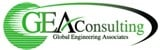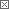GEA's Global HVAC Technology Blog Blog# GEA's Global HVAC Technology Blog

GEA's Global HVAC Technology Blog covers a range of topics including:

• Core HVAC Technologies
• Technology & Patent Evaluation
• Manufacturing Technologies
• Product Quality Improvement
• Materials/Failures/Corrosion
• Product/Technology Commercialization
• New Factory Design & Equipment

We'll draw upon our range of experts to provide comments, insights, technical articles and a little humor from time to time

We encourage your participation and feedback!

 Previous in Blog: NAR HVAC Market Expecting Strong Growth Next in Blog: Prefab Skyscrapers Reach for the Heavens Reshaping HVAC# Designing Flooded Evaporator Shell & Tube HXR's

Posted June 25, 2014 8:00 AM by larhere

In application, a water chiller must provide a specified flow rate of chilled water at specified entering & leaving water temperatures. A flooded evaporator has water on the tube side & refrigerant on the shell side. The unit compressor capacity and power consumption are functions of the refrigerant saturation temperature, among other operating parameters.

For the tube bundle, determine the flow areas and heat transfer areas.

Af=Nt*(π*Di2/4)/Np, tube side flow area, ft2

Ao=Nt*π*Do*L, shell side heat transfer area, ft2

Ai=Nt*π*Di*L, tube side heat transfer area, ft2

Aia= Nt*ai*L, tube side heat transfer area for fouling, ft2

ai=actual enhanced tube side area/ft, ft2/ft

B= Ao/ Ai

B2=Ao/Aia

Where

Nt=number of tubes

Np=number of tube passes

Di=inside tube diameter, ft

Do=outside tube diameter, ft

L=length of tube, ft

There are two equations that apply

Q=Uo*Ao*LMTD

and

Q=mw*cp*(Twi-Two)

Where

Q=Capacity, BTU/ Hr

Uo=Overall Heat Transfer Coefficient, BTU/ (Hr-Ft2-oF)

LMTD=Log Mean Temperature Difference, oF

LMTD=(Twi-Two)/ln((Twi-Ts)/ (Two-Ts))

mw=Mass Flow of water, Lb/Hr

cp=Specific heat of water, BTU/(Lb-oF)

Ts=Refrigerant saturation temperature, oF

Twi=Entering Water Temperature, oF

Two=Leaving Water Temperature, oF

The overall heat transfer coefficient is a function of the refrigerant side coefficient, the tube metal resistance, the water side coefficient and the fouling resistance.

Uo=1/((1/ho')+(B/hi)+(B2*Rf))

Where

ho'=refrigerant side heat transfer coefficient, BTU/(hr-ft2-oF) (determined by test)

Note: The metal wall resistance is commonly included with the refrigerant side heat transfer coefficient. No fouling resistance allowance is needed on the refrigerant side.

hi=water side heat transfer coefficient, BTU/(hr-ft2-oF) (determined by test)

Rf=water side fouling resistance, (hr-ft2-oF)/BTU (specified by customer or rating standard)

A frequent engineering calculation is to determine the refrigerant saturation given the required capacity, the water flow rate and the leaving water temperature. The solution for Ts is as follows:

Using the two equations that determine Q

Uo*Ao*LMTD=mw*cp*(Twi-Two)

Substituting the equation for LMTD

Uo*Ao*(Twi-Two)/ln((Twi-Ts)/ (Two-Ts))=mw*cp*(Twi-Two)

Uo*Ao/ln((Twi-Ts)/ (Two-Ts))=mw*cp

ln((Twi-Ts)/ (Two-Ts))= Uo*Ao/mw*cp

Define

C= Uo*Ao/mw*cp

Then

ln((Twi-Ts)/ (Two-Ts))=C

(Twi-Ts)/ (Two-Ts)=eC

Twi-Ts=eC*Two- eC *Ts

eC *Ts -Ts=eC*Two- Twi

Ts=(eC*Two- Twi)/( eC -1)

Designers perform iterative analyses with the aid of spreadsheets or computer programs to design optimum heat exchangers to meet the required duty.

Editor's Note: CR4 would like to thank James Larson, GEA Consulting Associates, for contributing this blog entry.

Interested in this topic? By joining CR4 you can "subscribe" to